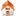# Spatiospectral concentration on a sphere

## Mark A. Wieczorek

### Département de Géophysique Spatiale et Planétaire Institut de Physique du Globe de Paris 94701 St. Maur-des-Fossés, France

SIAM Review, 48, (3), 2006, 504-536, doi:10.1137/S0036144504445765
 Cover Commentary Praise Related work Reprint Software Publications

#### Figures

1. Figure 01 Sketch illustrating the geometry of the spherical concentration problem.
2. Figure 02 Spectral eigensolutions to the problem of concentrating a spacelimited function within a spectral band.
3. Figure 03 Spatial eigensolutions to the problem of concentrating a bandlimited spherical harmonic expansion to a circular polar cap.
4. Figure 04 Eigenvalue spectra of the concentration problem to a circular polar cap, with partial Shannon numbers. (not included in paper)
5. Figure 05 Ranked eigenvalues of the concentration to circular polar caps for a fixed bandwidth.
6. Figure 06 Cumulative space-domain energy of the tapers for the spherical cap problem.
7. Figure 07 Ranked Grünbaum eigenvalues for L=18 and different concentration colatitudes. (not included in paper)
8. Figure 08 Grünbaum eigenfunctions for high-degree spherical caps. (not included in paper)
9. Figure 09 First and last four of Grünbaum eigenfunctions of the concentration with L=18 and radius 40°.
10. Figure 10 Eigenfunctions of the concentration to a circular polar cap of radius 40° for a fixed bandwidth of L= 18 in decreasing order of concentration.
11. Figure 11 Eigenvalue structure for the problems of localizing to different continents, for different values of the bandwidth L.
12. Figure 12 Concentrating Australia with bandlimited spherical harmonics (L=18).
13. Figure 13 Concentrating North America with bandlimited spherical harmonics (L=18) (not included in paper).
14. Figure 14 Concentrating Africa with bandlimited spherical harmonics (L=18).
15. Figure 15 Progressive buildup of energy by adding the square of the continental tapers weighted by their eigenvalue.
16. Figure 16 Comparison of several exact scaled kernels (grey) with their asymptotic approximation (black). (not included in paper)
17. Figure 17 Approximate number of significant eigenvalues given by the exact expression versus the asymptotic results (not included in paper).
18. Figure 18 Comparison of scaled spatial eigenfunctions (not included in paper).
19. Figure 19 Comparison of scaled spectral eigenfunctions (not included in paper).

Frederik Simons